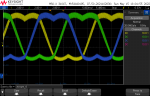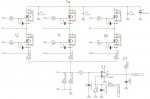# Need Help with Space Vector PWM

#### q747

I'm building a board to control a three-phase PMSM motor. I believe I have most of the control code implemented and working, except that my SVPWM algorithm generates a DC offset of 8v. I'm not sure what causes this and would like to eliminate it. As a comparison, I also implemented a straight PWM implementation that generates sine waves based on a lookup table, and looking at its output on an oscilloscope, the three sine waves are 120 degrees out of phase (as expected) and go down to 0v and up to close to the DC rail voltage. The signals generated by my SVPWM implementation look like textbook examples of SVPWM when I look at them on a scope (see attached scope shot), except that the center of the signals sit right at 8v. If I increase of decrease or decrease Iq, the span of the waves increases or decreases, but it's always centered on 8v. I'm not sure why this is--I'm not an expert on control theory or motor control--and I assume I need to get rid of this offset.

Can anyone help me understand the cause of the 8v DC offset and how to get rid of it? (assuming I do want to get rid of it.)

My SVPWM code is below. Input parameters are:

alpha, beta : converted from Iq, Id, and the rotor electrical angle using the inverse Park transform
theta: rotor electrical angle in radians
Vdc: DC rail in volts (12v in this case)
T: value in the period register of the timers used to generate PWM
Tu, Tv, Tw: outputs that are put into the PWM timer compare registers

The thing I'm most unsure about here is the calculation of the modulation index, which is used later to scale the PWM on times. Is my calculation of it correct?

Note: This code isn't optimized yet.

void svpwm(float alpha, float beta, float theta, float Vdc, float T, float *Tu, float *Tv, float *Tw)

{

float Vref, modulationIndex;
float Ta, Tb, T0, sinTheta;
uint32_t sector;

Vref = sqrtf((alpha * alpha) + (beta * beta));
modulationIndex = (Vref * PIOVER2) / Vdc;

/*
* Put theta in the range 0 - pi/3 (0-60 degrees) and determine which
* of the six sectors of the SV hexagon the angle lies.
*/
sector = 1;
while(theta > PIOVER3) {
theta -= PIOVER3;
sector++;
}

/*
* Ta and Tb are the on times the two sector endpoints used to synthesize the desired voltage.
* T0 is the time the (+++) and (---) states are active
* Tu, Tv, Tw are the times (in timer compare counts) the U, V, and W high-side MOSFETs are on.
*/
sinTheta = sinf(theta);
Ta = modulationIndex * (cosf(theta) - (ONEOVERSQRT3 * sinTheta));
Tb = modulationIndex * TWOOVERSQRT3 * sinTheta;
T0 = (T - (T * Ta) - (T * Tb)) / T;

switch(sector) {
case 1:
*Tu = T * (0.5f * T0);
*Tv = T * ((0.5f * T0) + Ta);
*Tw = T - (T * (0.5f * T0));
break;
case 2:
*Tu = T * ((0.5f * T0) + Tb);
*Tv = T * 0.5f * T0;
*Tw = T - (T * (0.5f * T0));
break;
case 3:
*Tu = T - (T * (0.5f * T0));
*Tv = T * (0.5f * T0);
*Tw = T * ((0.5f * T0) + Ta);
break;
case 4:
*Tu = T - (T * (0.5f * T0));
*Tv = T * ((0.5f * T0) + Tb);
*Tw = T * (0.5f * T0);
break;
case 5:
*Tu = T * ((0.5f * T0) + Ta);
*Tv = T - (T * (0.5f * T0));
*Tw = T * (0.5f * T0);
break;
case 6:
*Tu = T * (0.5f * T0);
*Tv = T - (T * (0.5f * T0));
*Tw = T * ((0.5f * T0) + Tb);
break;
}
}#### oneye14

Quite mystified at what you are doing ...

Mention of 3 phase and most of us are in the 400vAC range !
Forget the software because it appears to be providing results plus I couldn't help you anyway.

There is mention of MOSFET's but working at such low voltage, plus they are driving into virtually no load, reminds me more of a the o/p stage of an audio amplifier.
Are the MOSFET's working with a 'pulldown' resistor from 12vDC or 'pullup'resistor from 0vDC, or something else - a diagram would help here.

#### q747

This is for a motor control application of low-voltage (12-24v) PMSM motors. The hardware consists of six MOSFETs in three half-bridges with each half-bridge connected to one of the motor phases. Below is the schematic of the Infineon board I'm using to test this. The DC rail voltage on this board (VDD in the schematic below) is 16V.

When I generate three sine waves 120 degrees out of phase with each other, the sine waves on the scope do go all the way down to zero volts, as I would expect, but the signals generated by SVPWM always seem to have the 8V DC offset.The MOSFETs are driven by gate drivers, which in turn are driven by the PWM outputs of a microcontroller.

#### oneye14

The MOSFET's are working in 'symmetry' so the 2nd scope shot is what I would expect too.
I assume non of the resistors are faulty (RS4/5) or open circuit to cause drifting rather than overcurrent control; the only other thing to check is gate voltage with multimeter on DC (average or RMS) - each showing around 8vDC [H01/2/3]

Everything then would indicate towards a Software fault - nothing appears obviously wrong but not my area of expertise.

#### q747

Thanks, oneye14. I think I have the DC offset under control now. There's another thing I don't quite understand, but I'll create a separate post for that.

#### Carl

Looks like a third harmonic in the signal...should measure at the star point...to test point...
Star point is floating ground...and most likely not a balanced star point. I assume the caps are filtering your pwm ...so you can get sin like wave form
For a load rather connect some incandescent lamps...
If your making reference to uP GND and star point being the same...they are not the same in this case.
Also your scope should not be grounded...the proper way is to use an isolated probe...but there are other ways around this...but there are risks associated with not grounding the scope..expecially if the scope case is made of metal.